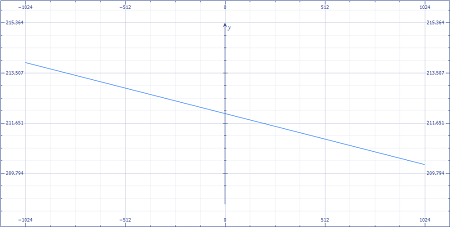# The temperature at which the water starts to boil is called its boiling point and is related to...

## Question:

The temperature at which the water starts to boil is called its boiling point and is related to the altitude. Water boils at {eq}212 ^\circ F {/eq} at sea level and {eq}193.6 ^\circ F {/eq} at an altitude of 10,000 feet.

1. Find a relationship of the form {eq}T = m x + b {/eq} where {eq}T {/eq} is in degrees Fahrenheit and {eq}x {/eq} is altitude in thousands of feet.

2. Find the boiling point at an altitude of 5000 feet.

3. Find the altitude if the boiling point is {eq}200 ^\circ F {/eq}.

4. Graph the equation in #1.

## Linear Function determination and calculation:

If two variables y and x varies linearly, then the relationship between the variables can be expressed as:

{eq}y = mx + b {/eq}

where

m is the slope of the line and can be found mathematically as:

{eq}m = \displaystyle \frac{y_2-y_1}{x_2-x_1} {/eq}

and

b is the y-intercept made by the line

Let x represent the altitude and T represent temperature

Given that;

{eq}\begin{align*} x_1 = 0 &\text{At sea level}\\ x_2 = 10,000 &\text{ Given data}\\ T_1 = 212 &\text{Boiling point of water at sea level}\\ T_2 = 193.6 &\text{Boiling point of water at 10,000 ft altitude}\\ \end{align*} {/eq}

1) To find the equation representing the relationship between temperature and altitude:

Given that temperature varies linearly with altitude

Therefore, we get;

{eq}y = mx + b {/eq}

Substituting {eq}x_1 = 0 {/eq} and {eq}T_1 = 212 {/eq} we get;

{eq}212 = m \times 0 + b\\ \therefore b = 212 {/eq}

For finding m, we know that

{eq}m = \displaystyle \frac{T_2-T_1}{x_2-x_1} {/eq}

Substituting the values we get;

{eq}m = \displaystyle \frac{193.6-212}{10000-0}\\ m = -0.00184 {/eq}

Therefore, the relationship between temperature and altitude can be expressed as:

{eq}T = -0.00184x + 212 {/eq}

2) To find the boiling point of water at 5000 feet

Substituting x=5000 in the equation for temperature we get;

{eq}T = -0.00184 \cdot 5000+ 212\\ \therefore T = 202.8 {/eq}

Therefore, water will start to boil at a temperature of 202.8 F at 5000 feet

3) To find the altitude at which water starts boiling at 200 F

Substituting the value of T=200 in the equation for temperature we get;

{eq}200 = -0.00184x + 212\\ x = \displaystyle \frac{200-212}{-0.00184}\\ \therefore x = 6521.73 {/eq}

Therefore, water boils at a temperature of 200 F at an altitude of 6521.73 ft

4) The graph of the equation is as follows: